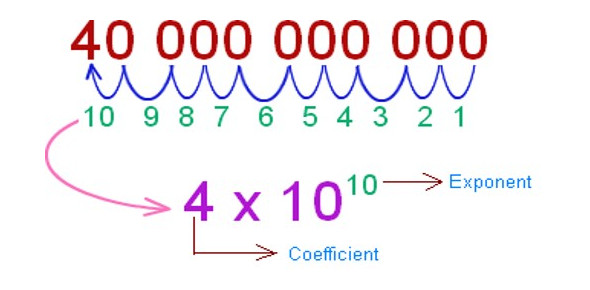# Scientific Notation Exam: Quiz!

Approved & Edited by ProProfs Editorial Team
The editorial team at ProProfs Quizzes consists of a select group of subject experts, trivia writers, and quiz masters who have authored over 10,000 quizzes taken by more than 100 million users. This team includes our in-house seasoned quiz moderators and subject matter experts. Our editorial experts, spread across the world, are rigorously trained using our comprehensive guidelines to ensure that you receive the highest quality quizzes.
| By Kyle Pearson
K
Kyle Pearson
Community Contributor
Quizzes Created: 36 | Total Attempts: 84,536
Questions: 10 | Attempts: 385Settings.

• 1.

### Is the following numbers in proper scientific notation? 23.2 x 102

• A.

Yes, this is proper scientific notation

• B.

No, this is not proper scientific notation

B. No, this is not proper scientific notation
Explanation
2.32 x 10^3

Rate this question:

• 2.

### Write the number 4500000 in scientific notation.

• A.

4.5 e6

• B.

45 e5

• C.

4.5 e-6

• D.

45,000

A. 4.5 e6
Explanation
The correct answer is 4.5 e6 because scientific notation is used to represent very large or very small numbers. In this case, 4500000 is written as 4.5 multiplied by 10 raised to the power of 6. This means that the decimal point is moved 6 places to the right, resulting in a number that is 4.5 million.

Rate this question:

• 3.

### Check ALL the following that are correctly in scientific notation:

• A.

93 x 10^2

• B.

0.93 x 10^2

• C.

9.3 x 10^2

• D.

4 x 10^13

• E.

9.4 x 10^-12

C. 9.3 x 10^2
D. 4 x 10^13
E. 9.4 x 10^-12
Explanation
The given answer correctly identifies the numbers that are written in scientific notation. Scientific notation is a way of expressing numbers as a product of a decimal number between 1 and 10, and a power of 10. In the answer, 9.3 x 10^2, 4 x 10^13, and 9.4 x 10^-12 are all written in this format. The other options, 93 x 10^2 and 0.93 x 10^2, are not in scientific notation because the decimal number is not between 1 and 10.

Rate this question:

• 4.

### What is the scientific notation for 3 500 000 000?

• A.

3.5 e9

• B.

3 e9

• C.

3.5 e8

• D.

3.500000000 e9

A. 3.5 e9
Explanation
The scientific notation is a way to express very large or very small numbers. In this case, the number 3,500,000,000 can be written as 3.5 multiplied by 10 raised to the power of 9, which is represented as 3.5e9.

Rate this question:

• 5.

### Write the number 0.0000045 in scientific notation.

• A.

4.5 e6

• B.

0.45 e

• C.

4.5 e-6

• D.

45,000

C. 4.5 e-6
Explanation
The given number, 0.0000045, can be expressed in scientific notation as 4.5 e-6. In scientific notation, the number is written in the form of a decimal number between 1 and 10 (in this case, 4.5) multiplied by a power of 10 (in this case, -6). The negative exponent indicates that the decimal point is moved 6 places to the left, resulting in a smaller number.

Rate this question:

• 6.

### Check ALL the ones that are correctly in scientific notation:

• A.

93 e2

• B.

0.93 e2

• C.

9.3 e2

• D.

4 e13

• E.

9.4 e-12

C. 9.3 e2
D. 4 e13
E. 9.4 e-12
Explanation
All of the given numbers are correctly written in scientific notation. 93 e2 is equivalent to 93 x 10^2, 0.93 e2 is equivalent to 0.93 x 10^2, 9.3 e2 is equivalent to 9.3 x 10^2, 4 e13 is equivalent to 4 x 10^13, and 9.4 e-12 is equivalent to 9.4 x 10^-12.

Rate this question:

• 7.

### Find the scientific form for 35.

• A.

3.5 e9

• B.

3 e9

• C.

3.5 e1

• D.

3.5 e-1

C. 3.5 e1
Explanation
The scientific form for a number is typically written in the form of a decimal number multiplied by a power of 10. In this case, the number 35 can be written as 3.5 multiplied by 10 raised to the power of 1. Therefore, the scientific form for 35 is 3.5 e1.

Rate this question:

• 8.

### What is the standard form for 9.4 e-3?

• A.

9400

• B.

0.0094

• C.

9.400

• D.

0.094

B. 0.0094
Explanation
The standard form for 9.4 e-3 is 0.0094. In scientific notation, the "e" represents "times ten raised to the power of." So, 9.4 e-3 means 9.4 times ten raised to the power of -3, which is equivalent to 0.0094.

Rate this question:

• 9.

### The E coli bacterium is about 5 x 10^-7 meters wide. A hair is about 1.7 x 10^-5 meters wide. Which is wider, the bacterium or the hair?

• A.

Bacterium

• B.

Hair

B. Hair
Explanation
The hair is wider than the bacterium. The given measurements indicate that the hair is approximately 34 times wider than the bacterium.

Rate this question:

• 10.

### Which number is smaller?          9.34e-4        or     3.2e-3

• A.

9.34e-4

• B.

3.2e-3

A. 9.34e-4
Explanation
The given question asks which number is smaller between 9.34e-4 and 3.2e-3. In scientific notation, both numbers are written in the form of a decimal multiplied by a power of 10. To compare the two numbers, we can ignore the power of 10 and focus on the decimal part. In this case, 9.34e-4 has a smaller decimal value compared to 3.2e-3. Therefore, 9.34e-4 is the smaller number.

Rate this question:

Related TopicsBack to top